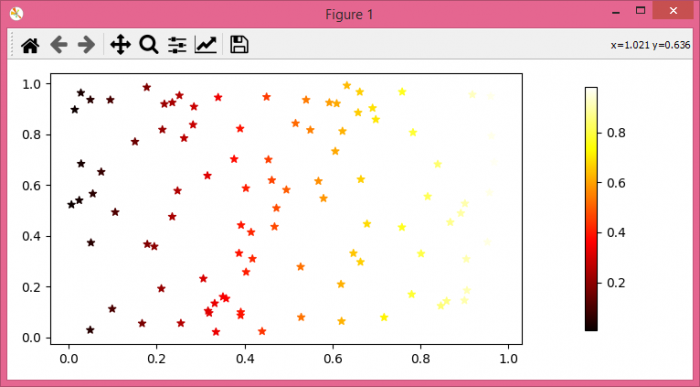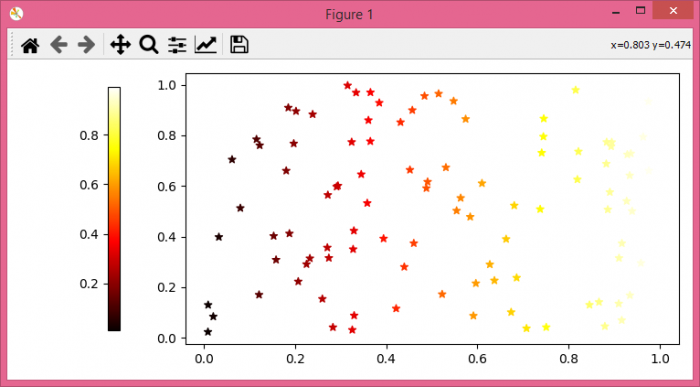# How to shift the colorbar position to right in matplotlib?

To shift the colorbar position to right in matplotlib, we can take the following steps −

## Steps

• Import numpy and matplotlib.

• Set the figure size and adjust the padding between and around the subplots.

• Initialize a variable N to store the number of sample data.

• Create x and y data points using numpy.

• Create a scatter plot using scatter() method with x and y data points.

• Add a colorbar to a plot, use the pad value for horizontal shift towards right or left.

• To display the figure, use show() method.

## Example

# Import numpy and matplotlib
import numpy as np
from matplotlib import pyplot as plt

# Set the figure size
plt.rcParams["figure.figsize"] = [7.50, 3.50]
plt.rcParams["figure.autolayout"] = True

N = 100

# Create x and y data points
x = np.random.rand(N)
y = np.random.rand(N)

# Scatter plot with x and y data points
s = plt.scatter(x, y, c=x, cmap='hot', marker='*')

# Display the plot
plt.show()

## Output

It will produce the following output −If you would like to set the colorbar to the left, then use the location parameter as shown below −

plt.colorbar(s, shrink=0.9, pad=0.1, location="left")


With this line in the code, we will have the output as follows −